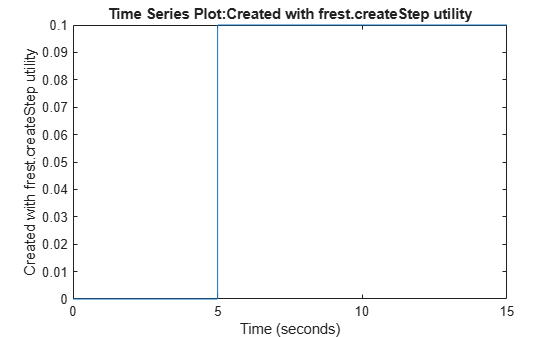# frest.createStep

Package: frest

Create step input signal

## Syntax

``input = frest.createStep(Name,Value)``

## Description

A step input signal has an initial value of `0` and transitions to a specified step size value after a specified step time. When performing frequency response estimation, step inputs are quick to simulate and can be useful as a first try when you do not have much knowledge about the system you are trying to estimate. However, the amplitude of the excitation decreases rapidly with increasing frequency. Therefore, step signals are best used to identify low-order plants where the slowest poles are dominant. Step inputs are not recommended for estimation across a wide range of frequencies.

When you use a step input signal for estimation, the frequencies returned in the estimated `frd` model depend on the length and sampling time of the signal. They are the frequencies obtained in the fast Fourier transform of the input signal (see the Algorithm section of `frestimate`).

For more information on input signals for frequency response estimation, see Estimation Input Signals.

example

````input = frest.createStep(Name,Value)` creates a step input signal for frequency response estimation using options specified using one or more name-value pair arguments.```

## Examples

collapse all

Create a step input signal with a default sample time and the following properties:

• Step time of `5` seconds

• Step size of `0.1`

• Total duration of `15` seconds

`input = frest.createStep('StepTime',5,'StepSize',0.1,'FinalTime',15)`
``` timeseries Common Properties: Name: 'Created with frest.createStep utility' Time: [15001x1 double] TimeInfo: tsdata.timemetadata Data: [15001x1 double] DataInfo: tsdata.datametadata ```

Plot the step signal.

`plot(input)`## Input Arguments

collapse all

### Name-Value Arguments

Specify optional pairs of arguments as `Name1=Value1,...,NameN=ValueN`, where `Name` is the argument name and `Value` is the corresponding value. Name-value arguments must appear after other arguments, but the order of the pairs does not matter.

Before R2021a, use commas to separate each name and value, and enclose `Name` in quotes.

Example: `'Ts',0.01` sets the input signal sample time to `0.01`

Sample time of the input signal in seconds, specified as the comma-separated pair `'Ts'`, followed by a positive scalar.

Step time when the input signal transitions from `0` to `StepSize`, specified as the comma-separated pair `'StepTime'` followed by a positive scalar.

`StepTime` must be less than `FinalTime`

Step size, specified as the comma-separated pair `'StepSize'` followed by a nonzero scalar. The input signal has value `StepSize` after `StepTime` seconds.

Input signal duration in seconds, specified as the comma-separated pair `'FinalTime'` followed by a positive scalar.

`FinalTime` must be greater than `StepTime`

## Output Arguments

collapse all

Step input signal for frequency response estimation, returned as a `timeseries` object.

To view a plot of your input signal, type `plot(input)`.

## Version History

Introduced in R2009b